# Arithmetic Series & Adding Numbers

in STEMGeeks2 months ago

Hi there. In this mathematics post, I would like to cover arithmetic series when it comes to adding numbers and arithmetic sequences.

Quicklatex.com is used for LaTeX math rendering.Pixabay Image Source

## Topics

• Arithmetic Sequences Basics
• Sum/Sigma Notation Basics
• Partial Sums For Arithmetic Sequences
• Examples

## Arithmetic Sequences Basics

An arithmetic sequence is a list of numbers where the next number is increased or decreased by the same number. Arithmetic sequences can be either increasing or decreasing.

One example of an increasing sequence is `4, 9, 14, 19, 24, 29, 34`. There is a constant increase of 5 to obtain the next number.

A decreasing sequence example includes `18, 8, -2, -12, -22, -32, -42`. The common difference here is negative 10.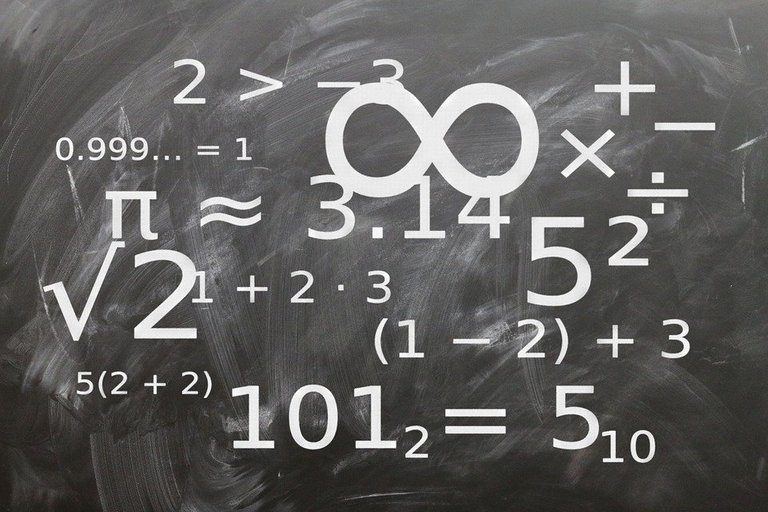Pixabay Image Source

## Sum/Sigma Notation Basics

Instead of writing the numbers from 1 to 100 being added together in the form of `1 + 2 + 3 + 4 + ... + 100`, you can use sum notation. This is a compact representation of adding numbers together from an arithmetic sequence.

Here is the representation in math notation.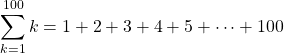The index variable `k` can be changed to a different letter such as `i` and `j`.

To represent a sum that does not start at 1 such as `5 + 6 + 7 + 8 + 9 + 10 + ... + 200` you would have this: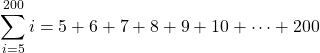Sums where the common difference is not equal to +1 can be represented with sum notation. Do note that sum/Sigma notation has its numbers going by one. If there is a common difference such as 3, a three would be added in front of the sum symbol. This would indicate that it is three times the sum or each number in the sum is multiplied by three. Here is one example: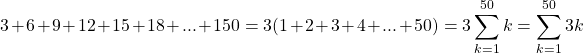The sum of negative even numbers from -2 to -20 can be represented as: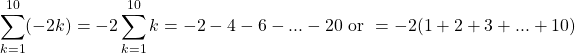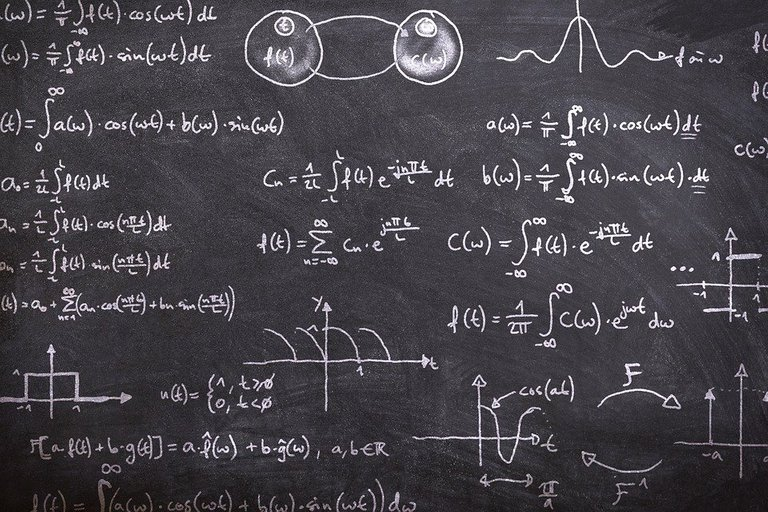Pixabay Image Source

## Partial Sums For Arithmetic Sequences

Once the concept of sum notation is grasped, partial sums involves the computation of the sum into a number. A formula can be used to compute a sum.

Let's revisit `1 + 2 + 3 + 4 + ... + 100`.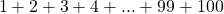Instead of adding from left to right with 1 all the way to 100, let's consider a smarter approach. Add the first number and last number together to obtain `1 + 100 = 101`. Now add the second number 2 and the second last number 99 to obtain 101. Continue with the 3 and 98 pair and work your way from the outside to the inside pair of numbers. All these number pairs have a sum of 101. How many number pairs are there that add up to 101? It is 50 which is half of 100.

For this example the sum of the counting numbers from 1 to 100 is equal to: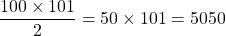General Formula

The example can be extended further with the use of a formula. Suppose there is a sum of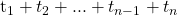where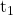is the first number in the series and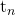is the last number in the series. The sum of these `n` numbers is given by the formula for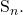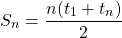Add the first and last numbers together first. Then multiply this sum by half of how ever many numbers there are in the sum.Pixabay Image Source

## Examples

That may be a lot of digest, let's add some numbers together with the formulas. Note that I do not have questions that involve finding numbers from an arithmetic sequence before using the partial sum formula. I do not cover sequences that start other than one nor index shifting.

Example One

What is the total of the even numbers starting from 2 to 222?

We have `2 + 4 + 6 + 8 + 10 + ... + 220 + 222`. This is the same as twice of `1 + 2 + 3 + 4 + 5 + 6 + ... + 110 + 111`. In math notation we can write: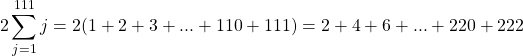As the question asks for the total of even numbers from 2 to 222 (inclusive), you can just use the partial sum formula.There are 111 numbers from 2 to 222 (inclusive). The first term `t_1` is 2 and the last term is 222. Use these numbers in the partial sum formula.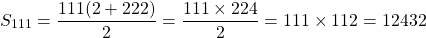Adding the even numbers starting from 2 to 222 gives 12 432.

Example Two

What is the total of the numbers from `-1, -2, -3, -4 to -55`?

The partial sum formula can be applied directly. There are 55 numbers.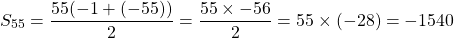Example Three - Algebraic Example

Determine the total of the values from `4x, 8x, 12x, 16x, ..., 996x to 1000x`.

If you want to represent this in sum notation, do make sure to common factor out the `4x` first.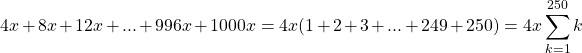The variable can be taken out of the sum as `x` is different from the sum index variable `k`.

Applying the partial sum formula gives: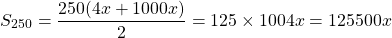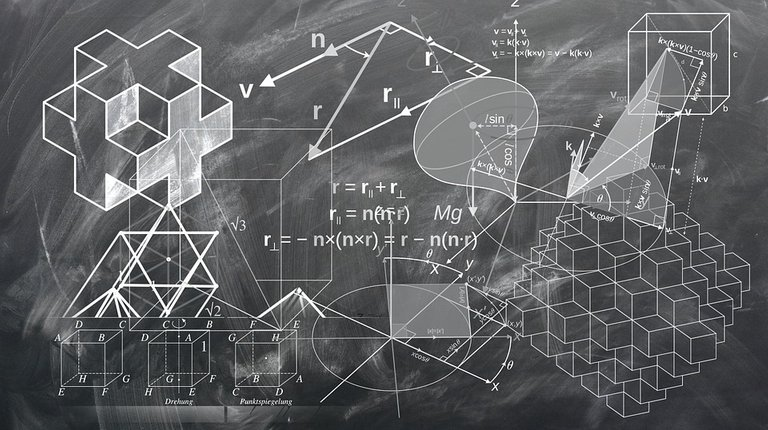Pixabay Image Source

Reference: